# Platonic & Archimedean Solids

Created by David Bronleewe 12/14/2001

These shapes are all of the Platonic and Archimedean Solids. A Platonic Solid is a 3 dimentional shape (polyhedra) that uses the same regular polygons (equilateral triangles, squares, etc.) as its surfaces. There are only 5 such shapes: tetrahedron, cube, octahedron, dodecahedron, and icosahedron.

Archimedean Solids can use 2 or more different types of regurlar polygons for its surfaces. But at each point it must have the same pattern of surfaces. There are in fact an infinite number of polyhedra that fit these requirements, but they fall into a different category. They are called prisms A(x, 4, 4) and antiprisms A(x, 3, 3, 3). There are only 13 that are not prisms or antiprisms and they are considered the 13 Archimedean Solids.

Each of these shapes is labeled with the types of surfaces it has. For example, the tetrahedron is labeled P(3, 3, 3) because at each point 3 triangles come together. And the cuboctahedron is labeled A(3, 4, 3, 4) because it is an Archimedean Solid and 2 triangles and 2 squares come together.

Also, if there is an * before the letter, like *P(5, 5, 5), then the object is 3 dimentional. The others are simply images. On the 3D ones, click and drag the object to rotate it and see it from any angle. If you would like to rotate it clockwise/counterclockwise, hold shift while you drage the mouse from side to side.

A dual is where the points on one shape match up with centers of the surfaces on the other shape. It is interesting to see how some of the Archimedean Solids are intermediate steps between the duals of the Platonic Solids.

Here the tetrahedron turns into its own dual:

 Tetrahedron*P(3, 3, 3)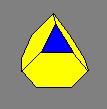Truncated TetrahedronA(3, 6, 6)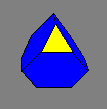Truncated TetrahedronA(3, 6, 6) Tetrahedron*P(3, 3, 3)

Here the cube turns into its dual, the octahedron:

 Cube*P(4, 4, 4) Truncated Cube*A(3, 8, 8) Cuboctahedron*A(3, 4, 3, 4) Truncated Octahedron*A(4, 6, 6) Octahedron*P(3, 3, 3, 3)

Here the dodecahedron turns into its dual, the icosahedron:

 Dodecahedron*P(5, 5, 5)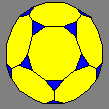Truncated DodecahedronA(3, 10, 10)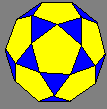IcosidodecahedronA(3, 5, 3, 5)Truncated IcosahedronA(5, 6, 6) Icosahedron*P(3, 3, 3, 3, 3)

These are the remaining Archimedean Solids: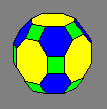Truncated CuboctahedronA(4, 6, 8)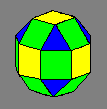RhomicuboctahedronA(3, 4, 4, 4)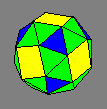Snub CubeA(3, 3, 3, 3, 4)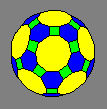Truncated IcosidodecahedronA(4, 6, 10)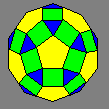RhombicosidodecahedronA(3, 4, 5, 4)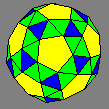Snub DodecahedronA(3, 3, 3, 3, 5)

Here is the source code I wrote to create the 3D objects. I wrote them as java applets using java. I wrote each of these files completely from scratch:

 Solid.java - This class is the main program and contains loads all the coordinates of the points. Double.java - This class stores both the integer and fractional part of a number. PointXYZ.java - This class stores information about the 3D points and has the routine that rotates the points. Surface.java - This class creates the surfaces on the polyhedra.

I originally wrote the code during the summer of 1999. I was just learning java and spent about 2 weeks perfecting it. I only programmed the cuboctahedron, not even knowing what it was called. I just did it because it was my favorite shape.

Now I've been going through and rewriting the code so I can add all the shapes. I've gotten all of the Platonic Solids and a few more of the Archimedean Solids. Athough even those haven't been easy. I'd say I've probablly put over 36 hours total in rewriting the code and calculating the the 3d points for each and every point on a solid. Some of the larger Archimedean Solids have far over a hundred points. So it might be some time before I get around to doing them. But for now, enjoy what I've got.

- David